##### Formula and Execution
 Cost Cost of an item. Margin The gross margin percentage is the profit divided by the selling price or revenue. Revenue It is the selling Price. Profit The gross profit is the difference between the cost to make a product and the selling price or revenue Markup The mark up percentage is the profit divided by the cost to make the product.

# Margin Calculator

In the rapidly changing world and the advancement in technology make it possible for us to do a work of hours in minutes and of minutes in seconds. The progress of technology helps us in every field of life, whether it is business or non-business field.

Accounting and finance are the two most essential components of the business; without them, business transactions are incomplete. The calculation in accounting is a quite hectic and challenging task, but with the advancement in technology, the accounting calculations are much easier and convenient. Now all these tough and challenging accounting calculations are a click away. Different types of software and calculators are developed by developers to make an accounting calculation easy and time-saving. One such calculator is a margin calculator.

Margin calculator is used to finding margin or profit as a result of the business transaction; companies and proprietors can both use this calculator to find their profit/loss as a result of the business transaction.

As the margin calculator is very simple so it is the best fit for the sole proprietor because transactions of a sole proprietor are of simple nature as compared to the company where the transactions are complex. This calculator is very helpful in finding out that whether the business transaction carried out by the sole proprietor or company is profitable or not. This calculator helps you in finding out the exact amount of profit/loss and the percentage of profit/Loss.

## How to Calculate Margin

Our Margin Calculator is very easy, simple and user friendly. This calculator operates in two modes, simple mode, and advance mode.

### Simple mode

In simple mode, the margin calculator only calculates profit and profit margin as shown in the below picture.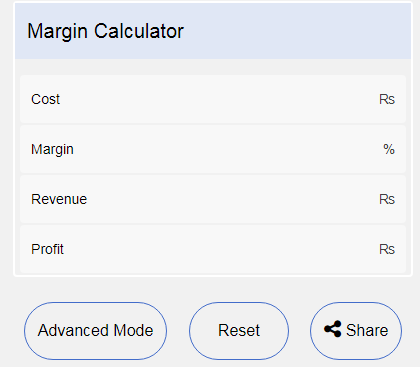There are four sections in simple mode, cost and revenues are the two most important sections because without putting values in these two sections, the other two sections (margin and profit) will give us no result. By putting values in cost and revenue sections, you will obtain values of profit and margin as shown below.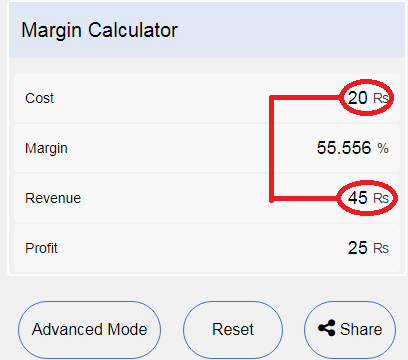By clicking on “reset”, the calculator will reset all the values to by default mode i.e. all the values in four sections will become zero. By clicking the “share” button, you can share the margin calculator on different social media sites with your friends and colleagues, etc. as shown in the below picture.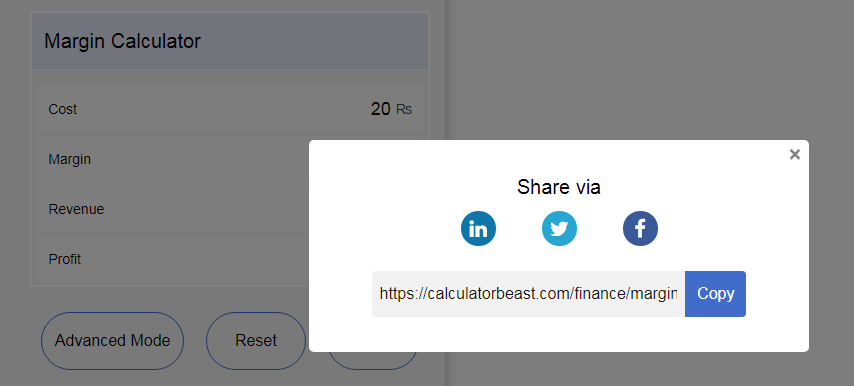There are no complications in advance mode. The use of the advance mode is effortless, straightforward. This mode is the same as a simple model with the only addition of “mark-up” as shown below.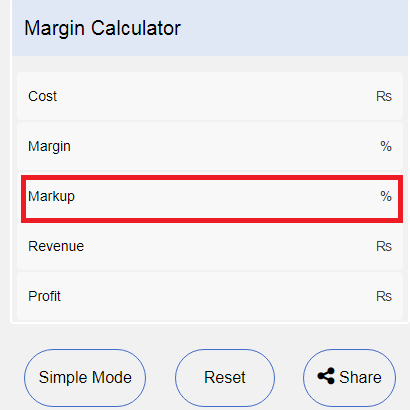There are five sections in advance mode, the most important sections are cost and revenue without which the other sections will give no values. By inserting values in cost and revenue sections, you will get the values in the margin, mark-up and profit sections as shown below.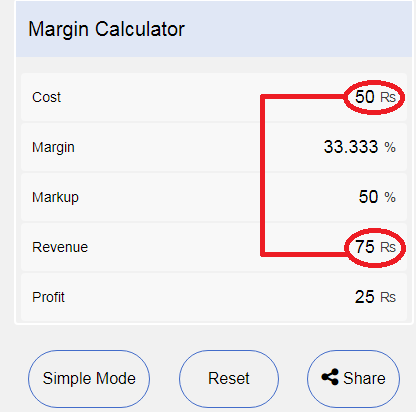You can reset the calculator by default mode by clicking the “reset” button while you can also share the calculator with your friends, colleagues and class fellows by clicking the share button.

## Explanation of the Margin Formulas used in calculations

We use three formulas in the margin calculator.

1. Profit/Loss formula
2. profit/Loss margin formula
3. Mark-up percentage

### Profit formula

The formula for profit calculation is (Revenue – cost), this will gives us a profit/Loss amount. Sometime the cost will be more and the revenue will be less, so in this case, business will generate no profit and the company will bear the loss. In the calculator, this amount will be located in the “profit” section.

For example revenue from the sale of the product is \$100 while the cost of the product is \$45 so by applying the above formula

Revenue = \$100

Cost = \$45

Profit = Revenue – cost

= 100-45 = 55

Profit= \$55

### Profit margin formula

In the margin formula, we represent the profit amount as a percentage of revenue. The formula for margin is (Profit/Revenue), this will give us a percentage of how much percent of the revenue is retained as a profit and how much is expense out in different accounting heads. The value of the profit margin will be located under the “margin” head in the margin calculator.

For example in the given example margin of the business will be 55%, by putting values in margin formula i.e.

Profit = \$55

Revenue = \$100

Margin = profit/Revenue

= 55/100= .55 or 55%

Margin = 55%

55%margin means that only 55% amount of the total revenue is saved as a profit and the remaining 45% is an expense out in different heads such as salaries of the employees, marketing expense, rent of the office, etc.

### Mark-up percentage

Mark-up means that how much amount additional amount is added to the cost of the product to get the desired profit margin. The formula for the calculation of mark-up is (profit/cost).In the margin calculator, the value of mark-up will be under the head “ mark-up” in advance mode. The simple mode does not calculate the mark-up percentage.

In the above example profit is \$55 while the cost of the product is \$45.Put values in mark-up formula.

Profit = \$ 55

Cost = \$ 45

Mark-up = 55/45= 1.22 or 122%

Selling price =45*1.22= 55

Selling price = 45+55= \$ 100.

Selling price =\$ 100

This means that the selling price of the product must be 1.22 times the cost of the product to get a \$55 profit.

## FAQS

### Question 1- How do you calculate margin?

Answer- When we deduct expenses from sales, the remaining amount is called margin. Margin is usually calculated in the percentage of sales. There are two types of margins:

Gross margin –When we deduct the cost of goods sold from the sales, the amount left is called gross margin/profit. Usually, gross margin is calculated as a percentage of sales i.e. Gross Margin/Sales = x%.

Net Margin- When we deduct all the expenses, including operating and non-operating expenses from the sales, the amount left is called net margin/profit.Net margin is calculated as a percentage of sales i.e. Net margin/sales = y%.

### Question 2- How you calculate a 30% or 40% margin?

Answer – To solve such a question where the selling price is not given, we divide the cost of the product with 1- the given margin i.e. {cost/ (1- margin)}. This formula will give us the selling price. For example, a product cost is \$ 30 and we want to generate a 30% margin from this particular product, put values in the above formula.

Cost = \$ 30

Margin = \$ 30%

Selling price =?

= cost/ 1-gross margin

= 30/1-.3

Selling price = \$ 43

This will give us a selling price of \$ 43. Profit, in this case, is \$ 13(43-30=13) so divided 43 by 13 and this will produce a margin of 30%.

In the case of a 40% margin, only change the margin value from 30% to 40% and apply the aforementioned method, it will give us the selling price on a 40% margin.

### Question 3 – How do you calculate margin on a calculator?

Answer – Margin calculation on the calculator is very simple and easy, just put the value of revenue and cost in the given margin calculator, it will give you the value of margin.

### Question 5 - What is the profit margin formula?

Answer - Profit margin is of two types as discussed in question 1, 1) Gross profit margin and 2) Net profit margin.

The formula of gross profit margin - Gross profit / Total sales

The formula of net profit margin - Net profit / Total sales

### Question 6 - What is a good profit margin?

Answer – This varies from industry to industry, for example, a profit margin of 15% is good for industry A but bad for industry B. Usually a margin above 25% is considered good for all types of industries and below 10% is considered below the average or bad profit margin.

### Question 7 - Is a 30% profit margin good?

Answer – A profit margin above 25% is considered good for any type of industry so 30% is a good profit margin.

### Question 8 - What markup is a 25% margin?

Answer – This will be done through the trial and error method. We will put random values of cost and revenue in margin calculator (advance mode), whichever values of revenue and cost give us a 25% margin, and we will check the markup percentage.

For example, if the cost is \$ 50 and revenue is \$ 67 this will give us a markup percentage of 34% as calculated below.

Cost = \$ 50

Revenue = \$ 67

Profit = \$ 17

Gross margin =17/ 50 = 25%

Markup percentage = 17/50 = 34%

Markup = 34%

### Question 9 - What is a 100% profit margin?

Answer - The formula for profit margin is (profit/sale)*100. By inserting values in this formula, we can derive any percentage.

### Question 10 – What is the profit margin percentage?

Answer – When we deduct expenses from sales, this will gives us profit, when we divide the profit by the sales this will give us a profit margin percentage.

For example, company A generates a sales of \$ 100, the cost of the sales was \$ 55. By deducting the cost from the sales this will give us profit i.e.

Sales = \$ 100

Cost = \$ 55

Profit= sales – cost

= 100- 55 = \$ 45

Profit = \$ 45

Dividing this profit by sales will give us a profit margin percentage.

Sales = 100

Profit = 45

Profit margin = 45/100 = .45 or 45%

Profit margin = 45%

### Question 11 - What is the difference between markup and profit margin?

Answer - Both of them give us the same result but in a different way.

Profit margin: Sales minus cost is called profit margin. In this case, selling price and cost of the product are given and we only derive profit margin by deducting the cost of the product from the sales. For example, the selling price of product x is \$ 60 and the cost of the product x is \$ 25 by deducting the cost from the selling price we will get profit.

Sale price = 60

Cost = 35

Profit = 60-25 = \$ 35

Profit = \$ 35

Profit margin is presented as a percentage of sales so

Sales =\$ 60

Profit = \$ 35

Profit margin = 35/60 = .58 or 58%

Profit margin = 58%

Markup: Markup is calculated as a percentage of cost i.e. (cost/1-profit).In markup, the cost of the product and the markup percentage is predetermined while selling price is unknown. Let suppose in the above scenario the selling price is unknown and the cost of the product and markup percentage is known quantities. To find the selling price we will use the formula (cost/1-markup percentage). Cost and markup percentage is already given so by putting values in the above formula we will get selling price i.e.

Cost = \$ 25

Profit margin = 58\$

Selling price = 25/ 1-58%= \$ 60

Selling price = \$ 60

The main difference between the two is that in profit margin the margin percentage is an unknown while selling price and product cost are known quantities. In the case of markup, the product cost and markup percentage are known quantities while selling price is unknown quantities.

### Question 13 – What is profit markup?

Answer – Profit markup is the percentage of sales. The formula of profit markup/margin is (profit/sales). We have discussed this in detail in question 2 and question 11 so you can refer to these questions for understanding profit markup/margin.

### Question 14 - What is the net profit margin ratio?

Answer – When we deduct all the operating and non-operating expenses from the gross profit, this gives us net profit. While dividing net profit by sales will gives us a net profit margin. The formula of net profit margin is (Net profit/ sales).

For example

Sales = \$ 100

Cost = \$ 50

Gross profit = \$ 50

Operating expenses = \$ 15

Non-operating expenses = \$ 10

Net profit = 50-15-10= \$ 25

Net profit = \$ 25

Net profit margin = 25/100= 0.25 or 25%

Net profit margin = 25%

### Question 15 - What happens if the net profit margin increases?

Answer – If the net profit margin increases it gives an indication that

- Sales of the business increase

- Operating expenses of the business decreases

Sales of the business increase: The increase in sales depends on the effectiveness of the marketing department that how effectively the marketing department is using their available resources if the increase in sales occurs it means that the marketing department is using all the resources effectively and efficiently.

The decrease in operating expenses: Marketing and administrative expenses are included in the operating expenses, they are directly related to the operation of the business that’s why they are called operating expenses. Decreases in operating expenses and at the same time increase in sales are the primary purpose of any business. If the operating expenses decreases and sales increase it means that business is cost-effective, streamlined and the system is well structured and is using all the resources to the best of their abilities.

### Question 16 – What is the net profit margin used for?

Answer- Investors usually use the net profit margin ratio for investment decision making in a particular company. If an investor is interested in investing in a particular company, he/she will first check the net profit margin ratio of the company. If this ratio is good then the investor will go ahead with the investment planning; otherwise he/she will turn to other companies with a higher net profit margin ratio.